• 十进制整数转换二进制采用“除2倒取余”，十进制小数转换二进制小数采用“乘2取整”。 例题： 135D = __ B 解析：如下图所示，将135除以2，得余数，直到不能整除，然后再将余数从下至上倒取。得到结果：1000 ...
1.十进制转R进制
1.1 十进制转二进制
十进制整数转二进制
十进制整数转换成二进制采用“除2倒取余”，十进制小数转换成二进制小数采用“乘2取整”。
例题： 135D = ______ B
**解析：**如下图所示，将135除以2，得余数，直到不能整除，然后再将余数从下至上倒取。得到结果：1000 0111B.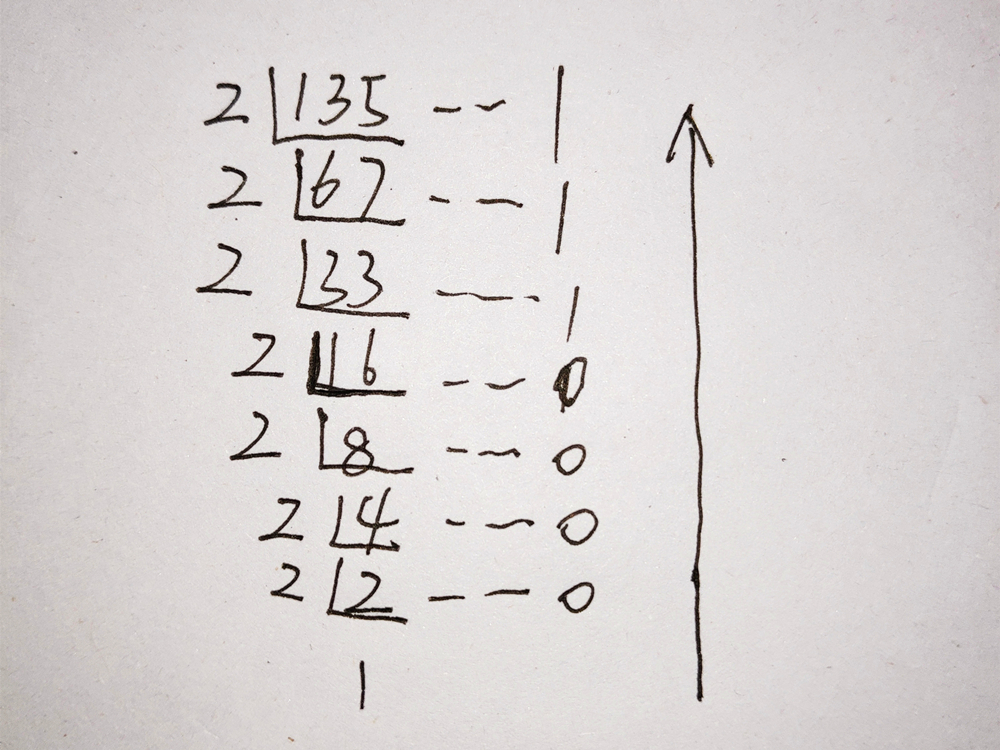图1.十进制整数转二进制
十进制小数转二进制
十进制小数转换成二进制小数采用 “乘2取整，顺序排列” 法。
具体做法是：
用2乘十进制小数，可以得到积，将积的整数部分取出，再用2乘余下的小数 部分，又得到一个积，再将积的整数部分取出，如此进行，直到积中的小数部分为零，或者达到所要求的精度为止。
然后把取出的整数部分按顺序排列起来，先取的整数作为二进制小数的高位有效位，后取的整数作为低位有效位。
例题： 0.68D = ______ B（精确到小数点后5位）
**解析：**如下图所示，0.68乘以2，取整，然后再将小数乘以2，取整，直到达到题目要求精度。得到结果：0.10101B.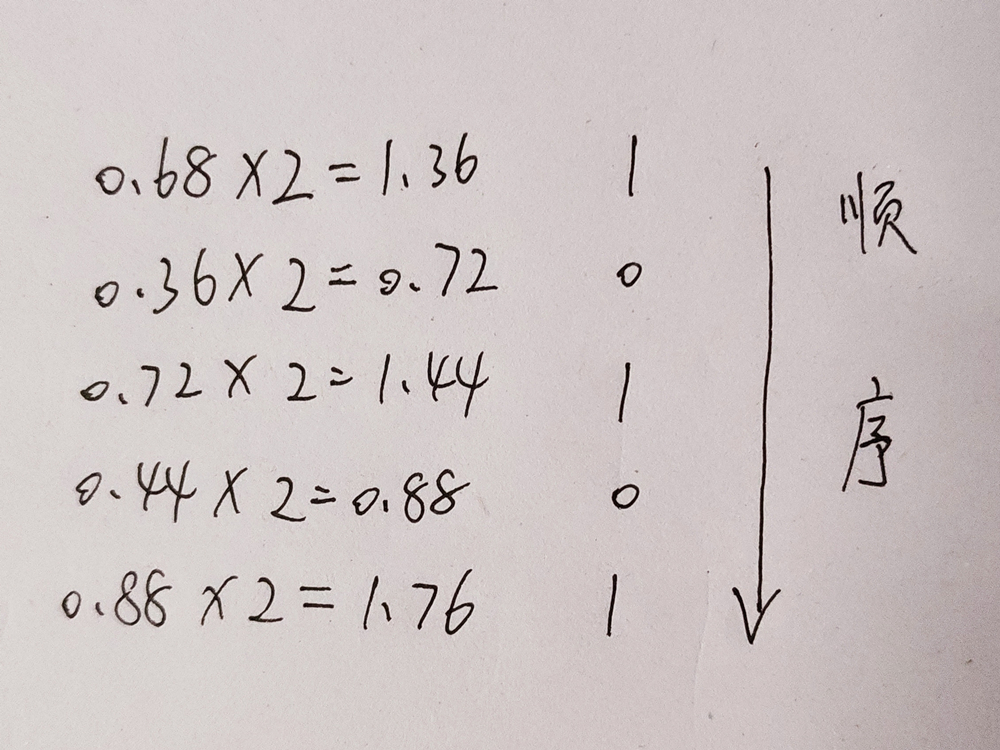图2.十进制小数转二进制
1.2 十进制转八进制
思路和十进制转二进制一样，参考如下例题：
例题： 10.68D = ______ Q（精确到小数点后3位）
**解析：**如下图所示，整数部分除以8取余数，直到无法整除。小数部分0.68乘以8，取整，然后再将小数乘以8，取整，直到达到题目要求精度。得到结果：12.534Q.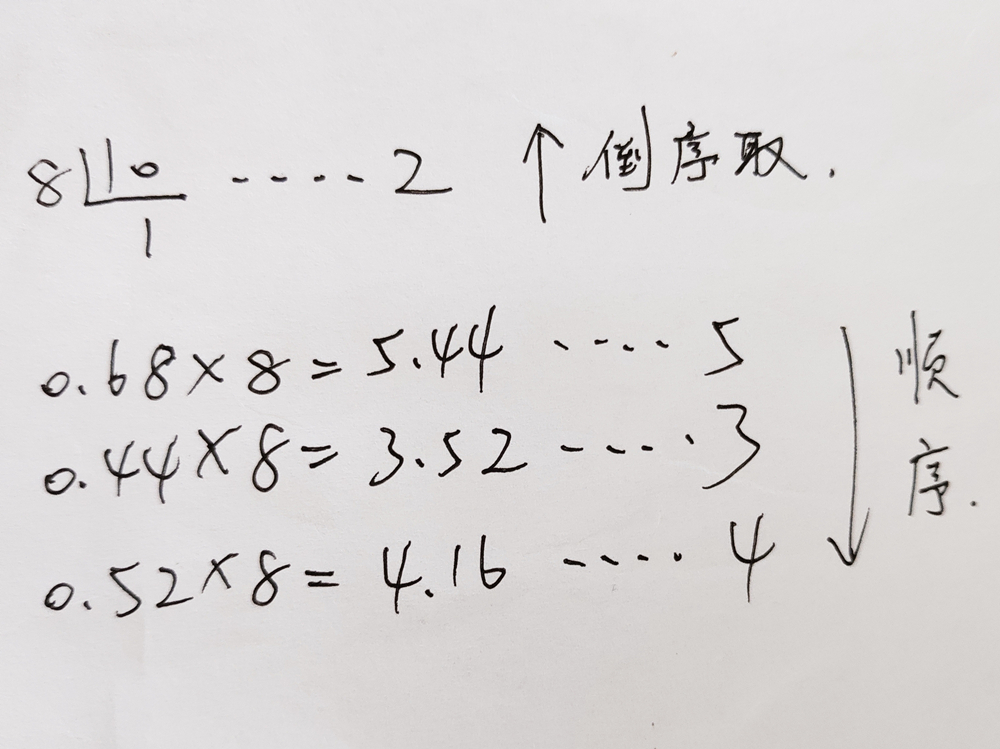图3.十进制转八进制
1.3 十进制转十六进制
思路和十进制转二进制一样，参考如下例题：
例题： 25.68D = ______ H（精确到小数点后3位）
**解析：**如下图所示，整数部分除以16取余数，直到无法整除。小数部分0.68乘以16，取整，然后再将小数乘以16，取整，直到达到题目要求精度。得到结果：19.ae1H.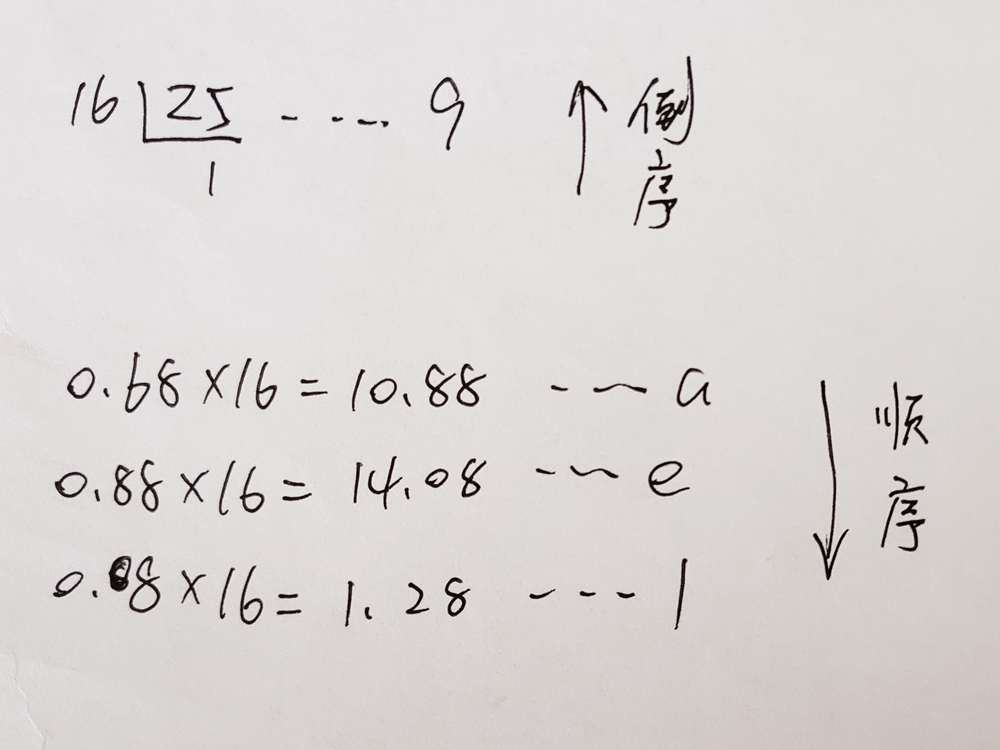图4.十进制转十六进制
# 2.R进制转十进制
## 2.1 二进制转十进制
**方法为：**把二进制数按权展开、相加即得十进制数。（具体用法如下图）
例题： 1001 0110B = ______ D
**解析：**如下图所示。得到结果：150D.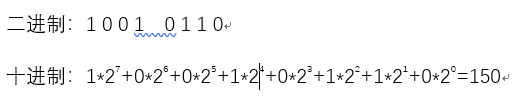图5.二进制转十进制
2.2 八进制转十进制
八进制转十进制的方法和二进制转十进制一样。
例题： 26Q = ______ D
**解析：**如下图所示。得到结果：22D.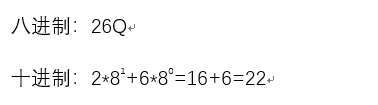图6.八进制转十进制
2.3 十六进制转十进制
例题： 23daH = ______ D
**解析：**如下图所示。得到结果：9178D.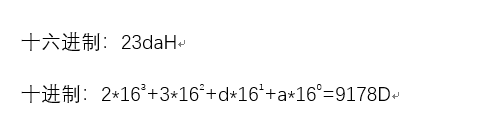图7.十六进制转十进制
3.二进制转八进制
二进制转换成八进制的方法是，取三合一法，即从二进制的小数点为分界点，向左（或向右）每三位取成一位。
例题： 1010 0100B = ____Q
**解析：**计算过程如下图所示。得到结果：244Q.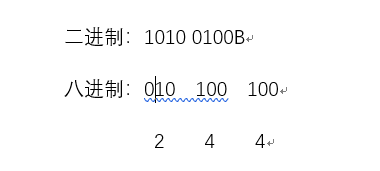图8.二进制转八进制
4.二进制转十六进制
二进制转换成八进制的方法是，取四合一法，即从二进制的小数点为分界点，向左（或向右）每四位取成一位。
例题： 1010 0100B = ____H
**解析：**计算过程如下图所示。得到结果：a4H.图9.二进制转十六进制
5.工欲善其事，必先利其器
下面的表格是8位二进制所对应的十进制数值，对进制转换以及类似题目的理解非常有用：

1
1
1
1
1
1
1
1
B

128
64
32
16
8
4
2
1
D

注：B：二进制
D：十进制

例题： 135D = ______ B
**解析：**有了上面二进制对应十进制数值的表格，我们就可以将题目给的十进制135拆分为：128+7，再从表格中找到对应的数值，拼凑即可得到答案。
135D = 128D + 7D = 1000 0111B


展开全文计算机基础
• //题目：八进制转换为十进制 #include<stdio.h> #include<math.h> int main() { int i=0,n,tmp,sum=0; scanf("%d",&n); while(n) { tmp=n%10; n=n/10; sum+=tmp*pow(8,i); i++;...
基本思想:   八进制转十进制，二进制转十进制都一样的道理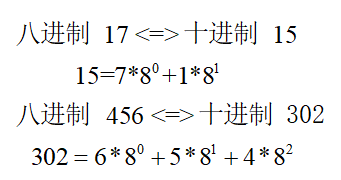八进制 --> 十进制
//【程序48】
//题目：八进制-->十进制
#include<stdio.h>
#include<math.h>
int main()
{
int i=0,n,tmp,sum=0;
scanf("%d",&n);
while(n)
{
tmp=n%10;
n=n/10;
sum+=tmp*pow(8,i);
i++;
}
printf("%d",sum);
return 0;
}

二进制 --> 十进制
#include<stdio.h> //二进制-->十进制
#include<math.h>
int main()
{
int i=0,n,tmp,sum=0;
scanf("%d",&n);
while(n)
{
tmp=n%10;
n=n/10;
sum+=tmp*pow(2,i);
i++;
}
printf("%d",sum);
return 0;
}

十进制 --> 二进制
#include<stdio.h>  //十进制-->二进制
int main()
{
int i=0,n,str;
scanf("%d",&n);
while(n)
{
str[i]=n%2;
n=n/2;
i++;
}
for(int j=i-1;j>=0;j--)
printf("%d",str[j]);
return 0;
}

十进制 --> 八进制
#include<stdio.h>  //十进制-->八进制
int main()
{
int i=0,n,str;
scanf("%d",&n);
while(n)
{
str[i]=n%8;
n=n/8;
i++;
}
for(int j=i-1;j>=0;j--)
printf("%d",str[j]);
return 0;
}

十六进制 --> 十进制
#include<stdio.h> //十六进制-->十进制 eg. (十六进制)AB-->171(十进制)
#include<math.h>                                  //ABCD-->43981
int main()
{
int i,j,k=0;
long int sum=0,tmp;
char str,a;
gets(str);
for(i=0;str[i]!='\0';i++)
{
if(str[i]>='0'&&str[i]<='9')
a[i]=str[i]-'0';
if(str[i]>='a'&&str[i]<='z')
a[i]=str[i]-'a'+1+9;
if(str[i]>='A'&&str[i]<='Z')
a[i]=str[i]-'A'+1+9;
}
for(j=i-1;j>=0;j--)
{
tmp=a[j]*pow(16,k);
sum+=tmp;
k++;
}
printf("%ld",sum);
return 0;
}
//#include<stdio.h>         //方法二：参看大佬的解法
//int main()                //16-->10进制
//{
//	int num = 0;
//    printf("输入一个十六进制数: ");
//    scanf("%x", &num);
//    printf("十六进制数 %x 转换为十进制为 %d\n", num, num);
//	return 0;
//}

十进制 --> 十六进制
#include<stdio.h>
int main()
{
int n,i=0,j;
char ten,str="0123456789ABCDEF";  //10进制-->16进制
scanf("%d",&n);                           //46-->2E; 59-->3B
while(n)                                  //46= 2*16^1 + 14*16^0
{
ten[i++]=str[n%16];
n=n/16;
}
for(j=i-1;j>=0;j--)
printf("%c",ten[j]);
return 0;
}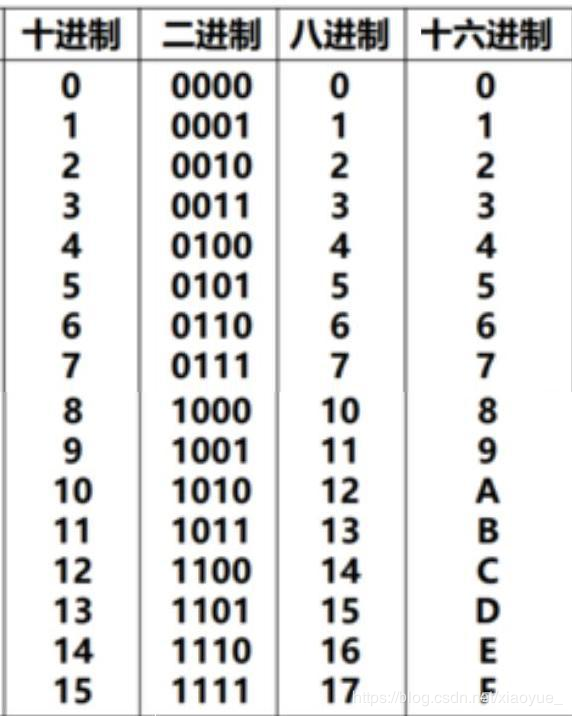展开全文c语言
• 十进制转换为十六进制，也就是 采用 除k取余法 ，直接让10处以16，再对 数据如果小于10和大于10进行处理，代码如下： #include<iostream> #include<string> using namespace std; int main() { ...
十进制转换为十六进制，也就是 采用 除k取余法 ，直接让10处以16，再对 数据如果小于10和大于10进行处理，代码如下：

#include<iostream>
#include<string>
using namespace std;
int main()
{
long a;//输入的数
int y=0;//循环中的余数
string s="";//输出的结果
cin>>a;
if(a==0)//0比较特殊，单独处理
{
cout<<0;
return 0;
}

while(a>0)//大于0的数
{
y=a%16;  //求余
if(y<10)   //小于10的余数
s=char('0'+y)+s;  //利用字符的ascll码在字符串前面拼接
else
s=char('A'-10+y)+s;  //大于9的余数用ABCDE表示
a=a/16;
}
cout<<s;
return 0;
}

十六进制转换为10进制，采取的是 乘以16

#include<iostream>
#include<string>
#include<math.h>
using namespace std;

int main()
{
string s;
int i=0,count,sum;
while(getline(cin,s))
{
int count=s.length();
sum=0;
for(i=count-1;i>=0;i--)//从十六进制个位开始，每位都转换成十进制
{
if(s[i]>='0'&&s[i]<='9')//数字字符的转换
{
sum+=(s[i]-48)*pow(16,count-i-1);
}
else if(s[i]>='A'&&s[i]<='F')//字母字符的转换
{
sum+=(s[i]-55)*pow(16,count-i-1);
}
}
cout<<sum;
}
}  

十进制 转换为 二进制，采取的是 除2取余法，其中把 2 换成其他小于10的数，可以变成相应的 其他进制

#include<iostream>

using namespace std;

int main(){
int a;
cin>>a;
int result=0;
int temp=a;
int j=1;
while(temp){
result=result+j*(temp%2);
temp=temp/2;
j=j*10;
}

cout<<result<<endl;

return 0;
} 


展开全文C++ 16进制
• java二进制转化为十进制Here you will get ... 在这里，您将获得在Java中将二进制转换为十进制的程序。 There are mainly two ways to convert a binary number to decimal number in Java. 在Java中，主要有两...java二进制转化为十进制Here you will get program to convert binary to decimal in Java.
在这里，您将获得在Java中将二进制转换为十进制的程序。
There are mainly two ways to convert a binary number to decimal number in Java.
在Java中，主要有两种将二进制数转换为十进制数的方法。
1. By using parseInt() method of Integer class.
2. By using user defined logic.
1.通过使用Integer类的parseInt()方法。 2.通过使用用户定义的逻辑。
用Java将二进制转换为十进制的程序 (Program to Convert Binary to Decimal in Java)
By using Integer.parseInt()
通过使用Integer.parseInt()
Integer.parseInt() method takes two arguments. First argument is a string and second argument is the base or radix in which we have to convert the number. The output is the integer represented by the string argument in the specified radix. Below is the program for it.
Integer.parseInt()方法采用两个参数。 第一个参数是一个字符串，第二个参数是我们必须在其中转换数字的基数或基数。 输出是由指定基数中的字符串参数表示的整数。 下面是它的程序。
import java.util.Scanner;

class BinaryToDecimal
{
public static void main(String args[])
{
Scanner s=new Scanner(System.in);

System.out.println("Enter a binary number:");

String n=s.nextLine();

System.out.println(Integer.parseInt(n,2));
}
}
Without using Integer.parseInt()
不使用Integer.parseInt()
In this method we have to define our own logic for converting binary number to decimal. The approach that we will use here is mentioned in below example.
在这种方法中，我们必须定义自己的逻辑，以将二进制数转换为十进制。 下面的示例中提到了我们将在此处使用的方法。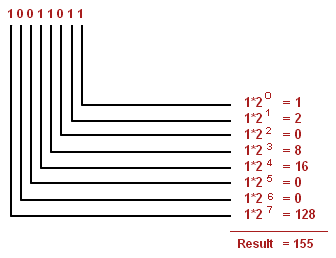Image Source
图片来源
The program that implements above approach is mentioned below.
下面介绍实现上述方法的程序。
import java.util.Scanner;

class BinaryToDecimal
{
public static void main(String args[])
{
Scanner s=new Scanner(System.in);

System.out.println("Enter a binary number:");
int n=s.nextInt();

int decimal=0,p=0;

while(n!=0)
{
decimal+=((n%10)*Math.pow(2,p));
n=n/10;
p++;
}

System.out.println(decimal);
}
}
Output
输出量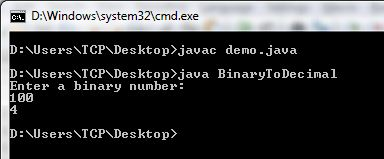If you found anything missing or incorrect in above programs then please mention it by commenting below.
如果您在上述程序中发现任何丢失或不正确的内容，请在下面的评论中提及。
翻译自: https://www.thecrazyprogrammer.com/2015/11/program-to-convert-binary-to-decimal-in-java.htmljava二进制转化为十进制
展开全文java python 编程语言 spring
• 1.十进制转换为二进制 #include using namespace std; int main(){  int N;  cout  int aa;  cin>>N;  int temp;  temp = N;  int num;  int i=0;  while(temp !=0)  {c++ integer input
• 二进制、八进制、十六进制转换为十进制 二进制、八进制和十六进制向十进制转换都非常容易，就是“按权相加”。所谓“权”，也即“位权”。 假设当前数字是N进制，那么： 对于整数部分，从右往左看，第i位的位权...
• c语言二进制转化为十进制Here you will get program to convert decimal to binary in C. 在这里，您将获得将C中的十进制转换为二进制的程序。 We can convert a decimal number into binary by repeatedly ...python java c++ c语言 算法
• 导语：在C语言中没有将其他进制的数直接输出为二进制数的工具或方法，输出八进制数可以用%o，输出十六进制可以用%x，输出为二进制就要我们自行解决了。下面给大家讲述一下如何编程实现将十进制转换二进制数...C语言 算法 CodeBlocks 入门
• 已知一个只包含 0 和 1 的二进制数，长度不大于 10 ，将其转换为十进制并输出。 输入描述 输入一个二进制整数n，其长度大于0且不大于10 输出描述 输出转换后的十进制数， 占一行 样例输入 110 样例...C++
• ## python将二进制转换为十进制

万次阅读 多人点赞 2017-05-15 08:49:20
例：将1110110转换为十进制数 解：先将其转换字符串，再使用int函数，指定进制转换为十进制。 print(int("100111",2))
• 二进制转换十进制二进制数第0位的权值是2的0次方，第1位的权值是2的1次方……所以，设有一个二进制数：101100100，转换为10进制为：356用横式计算(从右往左算)0×20+0×21+1×22+0×23+0×24+1×25+1×26+0×27+1×...
• 二进制 二进制就是计算机常用的进制，即逢二进一。例如：1010 八进制 八进制即逢八进一。例如：626 十进制 十进制就是我们在计算中常用的进制，所以就不再举例（即逢十进一） 十六进制 十六进制与其它进制...八进制 十六进制
• 解释：将一个十进制数除以二，得到的商再除以二，依此类推直到商等于一或零时为止，倒取将除得的余数，即换算为二进制数的结果 例如把52换算成二进制数，计算结果如图：   52除以2得到的余数依次：0、0、1、...
• * 文件名：二进制转换.c * 功能描述：把10进制数转换二进制数 * 编写人：王廷云 * 编写日期：2017-1-10 */ #include &amp;lt;stdio.h&amp;gt; #define NR (sizeof(int)*8) // 二进制位的个数 ...C语言
• 十进制小数转换二进制小数把十进制小数转换二进制小数所采用的规则是“乘以2取整数”。方法是用2乘以十进制纯小数，将其结果的整数部分去掉；再用2乘余下的纯小数部分，再去掉其结果的整数部分；如此继续下去，...
• 二进制转化为十进制用除法，就是除以100，然后除以10。假设43H是入口，出口是44H，45H，如果用44H的低四位存放十进制的百位，用45H的高四位和低四位分别存放十进制的十位和个位，应该怎么把分离出来的数分别存进高四...汇编语言
• 值得注意的是,存放二进制的数组...二进制转换为八进制是非常简单的,因为每三个二进制就是一位八进制.如果不足三位二进制则补0. 例如: 10101 –>010 101 –>25 1101 –> 001 101 –> 15 思路有了,接下来就是把思路转八进制 十六进制
• 在实际开发中，我们可能会...1、自定义的二进制字符串转十进制bintodec 思路：把二进制字符串从最高位（左边第一位）开始用商乘以2再加余数（该位的数字），如此循环，左边第一位的商肯定是0。 例如1111011转化成十...
• 十进制如何转二进制：将该数字不断除以2直到商为零，然后将余数由下至上依次写出，即可得到该数字的二进制表示。 以将数字21转化为二进制为例 当商为零时，将余数由下至上依次写出，即为21的二进制表示 #include<...算法 c++
• 二进制转换为十进制 1. 将十进制转换二进制： 思路：对十进制的数进行除2取余法： /** * 讲10 进制转化二进制 * @param de ：待转换的十进制 * @return ：转换后的二进制（string） */ public static ...java
• 二进制十进制代码截图 实现思路:就是调用递归函数,函数里面的变量i=i*2；从最后一位开始，越到前边i就会越大，刚好都是以2的倍数增长，所以满足二进制十进制条件(有的老师不让用全局变量，那就直接返回该整条...
• 十进制到任意进制的转换原理: 除积倒取余 十进制转换为十进制: 十进制转换为二进制: 60的二进制结果:111100 十进制转为八进制: 十进制转为十六进制:...Java
• #include <...//十进制转换为二进制 long long convertDecimalToBinary(int n); int main() { int n; printf("输入一个十进制数："); scanf("%d",&n); printf("十进制数%d 转换为二进制位 %lld\n...
• 本文介绍了python十进制二进制转换方法(含浮点数)，分享给大家，也给自己留个笔记，具体如下： 我终于写完了 ， 十进制二进制的小数部分卡了我将近一个小时 上代码 #-*- coding: utf-8 from decimal ...
• import java.math.BigInteger; /** * @author 进制转换 * */ public class cons { ... @SuppressWarnings("unused") ... // TODO 十六进制转换十进制 long result = 0; for(int i=(serial.length-1);i>=0;i-字符串 java
• 二进制转换为十进制的简便方法。 原来方法： 从最后一位开始算，依次列为第0、1、2...位 第n位的数（0或1）乘以2的n次方 得到的结果相加就是答案 例如: 01101011转十进制: 第0位:1乘2的0次方=1  1乘2的1次方...
• 一.本文所涉及的内容... 二进制十进制 八进制 → 十进制 十六进制 → 十进制十进制） → （二、八、十六进制） 十进制二进制 十进制 → 八进制 十进制 → 十六进制 （...基础
• 二进制、八进制、十六进制转换为十进制 二进制、八进制和十六进制向十进制转换都非常容易，就是“按权相加”。所谓“权”，也即“位权”。 假设当前数字是 N 进制，那么： 对于整数部分，从右往左看，第 i 位的...八进制 十六进制
• 1、使用java内部提供的方法，直接进行api的调用 public static void binaryTodecimal2(int n) { String res = Integer.toBinaryString(n); System.out.println(res);... // 将字符串转换为数字进行打印......